Next: Guiding Centre Motion Up: Charged Particle Motion Previous: Motion in Uniform Fields

# Method of Averaging

In many dynamical problems, the motion consists of a rapid oscillation superimposed on a slow secular drift. For such problems, the most efficient approach is to describe the evolution in terms of the average values of the dynamical variables. The method outlined below is adapted from a classic paper by Morozov and Solov'ev.Consider the equation of motion(43)

whereis a periodic function of its last argument, with period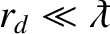, and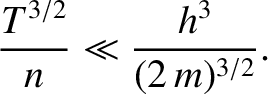(44)

Here, the small parameter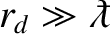characterizes the separation between the short oscillation periodand the time-scale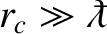for the slow secular evolution of the position''.

The basic idea of the averaging method is to treatandas distinct independent variables, and to look for solutions of the form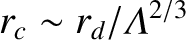which are periodic in. Thus, we replace Eq. (43) by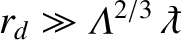(45)

and reserve Eq. (44) for substitution in the final result. The indeterminacy introduced by increasing the number of variables is lifted by the requirement of periodicity in. All of the secular drifts are thereby attributed to the-variable, whilst the oscillations are described entirely by the-variable.

Let us denote the-average ofby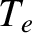, and seek a change of variables of the form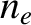(46)

Here,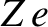is a periodic function ofwith vanishing mean. Thus,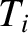(47)

wheredenotes the integral over a full period in.

The evolution ofis determined by substituting the expansions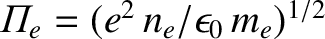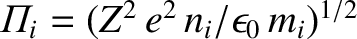(48)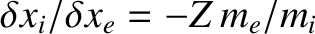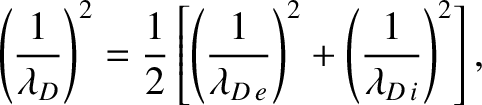(49)

into the equation of motion (45), and solving order by order in.

To lowest order, we obtain(50)

The solubility condition for this equation is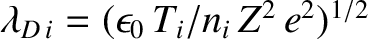(51)

Integrating the oscillating component of Eq. (50) yields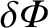(52)

To first order, we obtain(53)

The solubility condition for this equation yields(54)

The final result is obtained by combining Eqs. (51) and (54):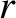(55)

Note thatin the above equation. Evidently, the secular motion of the guiding centre'' positionis determined to lowest order by the average of the force'', and to next order by the correlation between the oscillation in the position''and the oscillation in the spatial gradient of the force.''Next: Guiding Centre Motion Up: Charged Particle Motion Previous: Motion in Uniform Fields
Richard Fitzpatrick 2011-03-31